Custom SearchSUMMARY Before going on to the next chapter, study the below summary to be sure that you understand the important points of this chapter. THE ELECTROSTATIC FIELD - When a charged body is brought close to another charged body, the bodies either attract or repel one another. (If the charges are alike they repel; if the charges are opposite they attract). The field that causes this effect is called the ELECTROSTATIC FIELD. The amount by which two charges attract or repel each other depends upon the size of the charges and the distance between the charges. The electrostatic field (force between two charged bodies) may be represented by lines of force drawn perpendicular to the charged surfaces. If an electron is placed in the field, it will move toward the positive charge.CAPACITANCE - Capacitance is the property of a circuit which OPPOSES any CHANGE in the circuit VOLTAGE. The effect of capacitance may be seen in any circuit where the voltage is changing. Capacitance is usually defined as the ability of a circuit to store electrical energy. This energy is stored in an electrostatic field. The device used in an electrical circuit to store this charge (energy) is called a CAPACITOR. The basic unit of measurement of capacitance is the FARAD (F). A one-farad capacitor will store one coulomb of charge (energy) when a potential of one volt is applied across the capacitor plates. The farad is an enormously large unit of capacitance. More practical units are the microfarad (mF) or the picofarad (pF).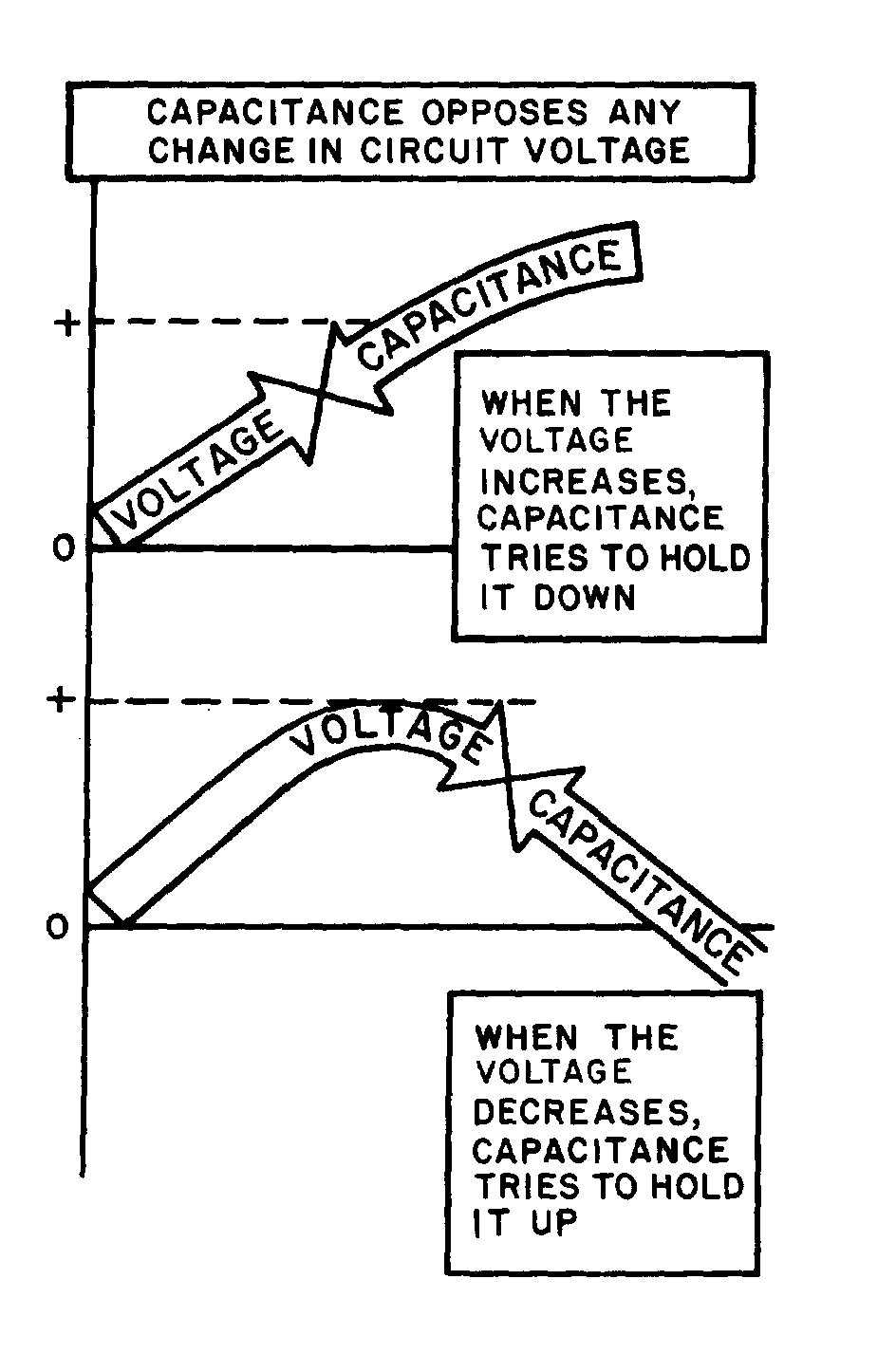CAPACITOR - A capacitor is a physical device consisting of two pieces of conducting material separated by an insulating material. This insulating material is referred to as the DIELECTRIC. Because the dielectric is an insulator, NO current flows through the capacitor. If the dielectric breaks down and becomes a conductor, the capacitor can no longer hold a charge and is useless. The ability of a dielectric to hold a charge without breaking down is referred to as the dielectric strength. The measure of the ability of the dielectric material to store energy is called the dielectric constant. The dielectric constant is a relative value based on 1.0 for a vacuum.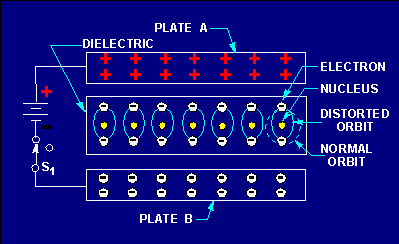CAPACITORS IN A DC CIRCUIT - When a capacitor is connected to the terminals of a battery, each plate of the capacitor becomes charged. The plate connected to the positive terminal loses electrons. Because this plate has a lack of electrons, it assumes a positive charge. The plate connected to the negative terminal gains electrons. Because the plate has an excess of electrons, it assumes a negative charge. This process continues until the charge across the plates equals the applied voltage. At this point current ceases to flow in the circuit. As long as nothing changes in the circuit, the capacitor will hold its charge and there will be no current in any part of the circuit. If the leads of the capacitor are now shorted together, current again flows in the circuit. Current will continue to flow until the charges on the two plates become equal. At this point, current ceases to flow. With a dc voltage source, current will flow in the circuit only long enough to charge (or discharge) the capacitor. Thus, a capacitor does NOT allow dc current to flow continuously in a circuit.FACTORS AFFECTING CAPACITANCE - There are three factors affecting capacitance. One factor is the area of the plate surfaces. Increasing the area of the plate increases the capacitance. Another factor is the amount of space between the plates. The closer the plates, the greater will be the electrostatic field. A greater electrostatic field causes a greater capacitance. The plate spacing is determined by the thickness of the dielectric. The third factor affecting capacitance is the dielectric constant. The value of the dielectric constant is dependent upon the type of dielectric used. WORKING VOLTAGE - The working voltage of a capacitor is the maximum voltage that can be steadily applied to the capacitor without the capacitor breaking down (shorting). The working voltage depends upon the type of material used as the dielectric (the dielectric constant) and the thickness of the dielectric. CAPACITOR LOSSES - Power losses in a capacitor are caused by dielectric leakage and dielectric hysteresis. Dielectric leakage loss is caused by the leakage current through the resistance in the dielectric. Although this resistance is extremely high, a small amount of current does flow. Dielectric hysteresis may be defined as an effect in a dielectric material similar to the hysteresis found in a magnetic material. RC TIME CONSTANT - The time required to charge a capacitor to 63.2 percent of the applied voltage, or to discharge the capacitor to 36.8 percent of its charge. The time constant (t) is equal to the product of the resistance and the capacitance. Expressed as a formula:where t is in seconds, R is in ohms, and C is in farads.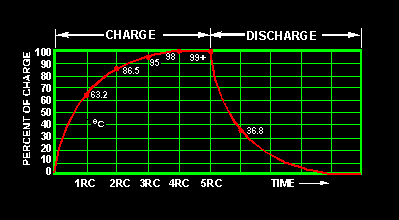CAPACITORS IN SERIES - The effect of wiring capacitors in series is to increase the distance between plates. This reduces the total capacitance of the circuit. Total capacitance for series connected capacitors may be computed by the formula: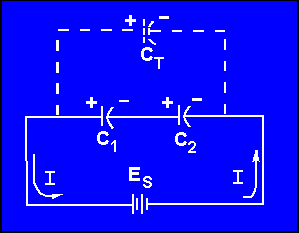If an electrical circuit contains only two series connected capacitors, CT may be computed using the following formula: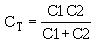CAPACITORS IN PARALLEL - The effect of wiring capacitors in parallel is to increase the plate area of the capacitors. Total capacitance (CT) may be found using the formula: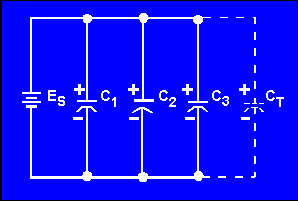TYPES OF CAPACITORS - Capacitors are manufactured in various forms and may be divided into two main classes-fixed capacitors and variable capacitors. A fixed capacitor is constructed to have a constant or fixed value of capacitance. A variable capacitor allows the capacitance to be varied or adjusted.Integrated Publishing, Inc. - A (SDVOSB) Service Disabled Veteran Owned Small Business